JEE  >  Basics of Trigonometry

# Basics of Trigonometry - Notes | Study Physics For JEE - JEE

 1 Crore+ students have signed up on EduRev. Have you?

Angle: Consider a fixed straight line OX. Let another straight line OA (called revolving line) be coinciding with OX rotate anticlockwise and takes the position OA, The angle is measured by the amount of revolution that the revolving line OA undergoes in passing from its initial position to final position.
From Figure given below, angle covered by revolving line OA is θ = ∠AOX.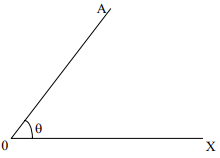An angle ∠AOX is +ve, if it is traced out in anticlockwise direction and ∠AOX is –ve, if it is traced out in clockwise direction

System of Measurement of an Angle

• Sexagesimal System
• Circular system

1. Sexagesimal System:
In this system
1 right angle = 90O (degrees)
1 degree = 60’ (minutes)
1 minute = 60’’ (seconds)

2. In circular system:
π Radians = 180° = 2 right angles
∴ 1 right. Angle = π/2 radians.

Let a particle moves from initial position A to the final position B along a circle of radius r as shown in figure.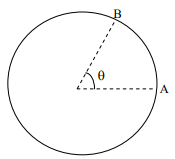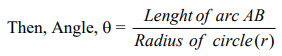If length of arc AB = radius of the circle (r) Then θ = 1 radian.

Radian: An angle subtended at the centre of a circle by an arc whose length is equal to the

When a body or a particle completes one rotation, then θ = 360° and distance travelled (circumference of a circle).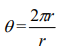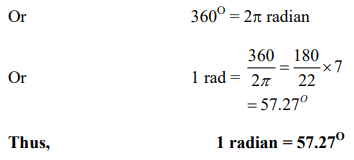Trigonometric Ratios

Consider triangle ONM in the four quadrants as shown below.
Consider two straight lines X'OX and Y'OY meeting at right angles in O. These two lines divide the plane into four equal parts called quadrants (figure given below).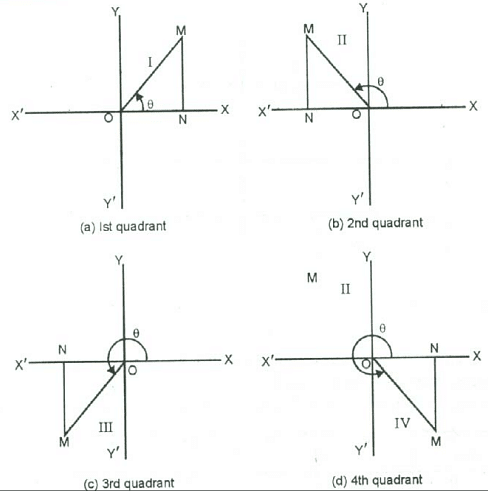Now XOY, YOX', X'OY' and Y'OX are called I, II, III, and IV quadrants respectively. ON is +ve if drawn to the right side of O and −ve if drawn to the left side of O. MN is +ve if drawn above X'OX and −ve if drawn below X'OX,
Trigonometric Ratios of an Angle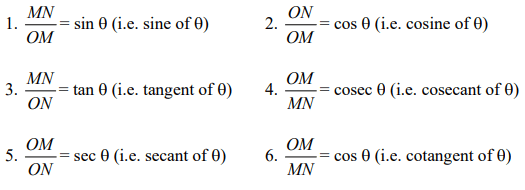These ratios are called trigonometric ratios.
Important relations: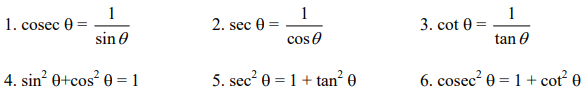Signs of trigonometric ratios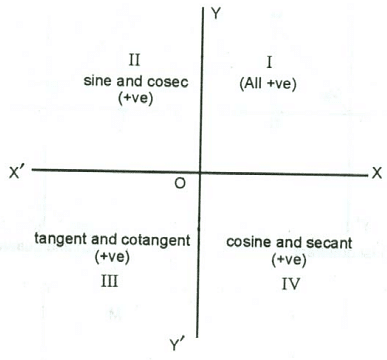The signs of various trigonometric ratios can be remembered from the above figure.

Trigonometric Ratios of Standard angles

The trigonometric ratios of standard angles are given in the following table: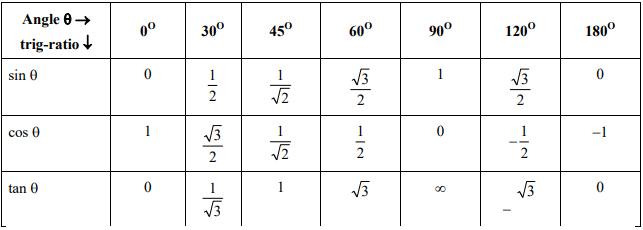Trigonometrical Ratios of Allied Angles

• (i) sin (−θ) = − sin θ (ii) cos (−θ) = cos θ (iii) tan (−θ) = − tan θ
• (i) sin (90°−θ) = cos θ (ii) cos (90°−θ) = sin θ (iii) tan (90°−θ) = cot θ
• (i) sin (90°+θ) = cos θ (ii) cos (90°+θ) = sin θ (iii) tan (90°+θ) = −cot θ
• (i) sin (180°−θ) = sin θ (ii) cos (180°−θ) = − cos θ (iii) tan (180°−θ) = − tan θ
• (i) sin (180°+θ) = −sin θ (ii) cos (180°+θ) = − cos θ (iii) tan (180°+θ) = − tan θ
• (i) sin (270°−θ) = − cos θ (ii) cos (270°−θ) = −sin θ (iii) tan (270°−θ) = cot θ
• (i) sin (270°+θ) = −cos θ (ii) cos (270°+θ) = sin θ (iii) tan (270°+θ) = −cot θ

Example:

Find the values of
(i) sin 270° (ii) sin 120° (iii) sin 120° (iv) tan (-30°)

(i) sin 270° = sin (180° + 90°) = − sin 90° = −1

(ii) cos 120° = cos (90° + 30°) = − sin 30° = −1/2

(iii) sin 120° = sin (90° + 30°) = cos 30° =√3/2

(iv) tan (−30°) = − tan 30° = - 1/√3

Some important Trigonometric Formulae

1. sin (A+B) = sin A cos B + cos A sin B
2. cos (A+B) = cos A cos B − sin A sin B
3. sin (A−B) = sin A cos B − cos A sin B
4. cos (A−B) = cos A cos B + sin A sin B
5. sin 2 A = 2 sin A cos A
6. sin (A+B) sin (A−B) = sin2 A − sin2 B
7. cos (A+B) cos (A−B) = cos2 A − sin2 B
8.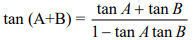9.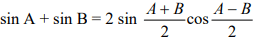10.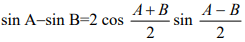11.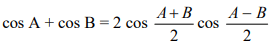12.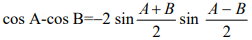13. cos2A = 1 − 2sin2A = 2 cos2A −1
14.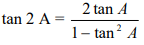15.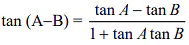16.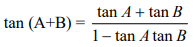The document Basics of Trigonometry - Notes | Study Physics For JEE - JEE is a part of the JEE Course Physics For JEE.
All you need of JEE at this link: JEE

## Physics For JEE

257 videos|633 docs|256 tests
 Use Code STAYHOME200 and get INR 200 additional OFF

## Physics For JEE

257 videos|633 docs|256 tests

Track your progress, build streaks, highlight & save important lessons and more!

,

,

,

,

,

,

,

,

,

,

,

,

,

,

,

,

,

,

,

,

,

;